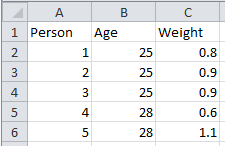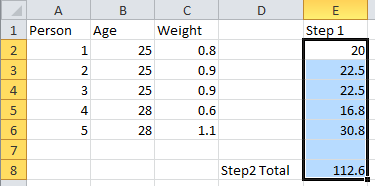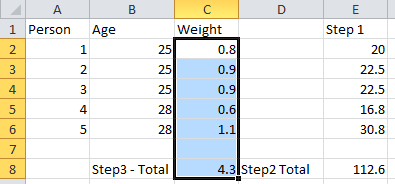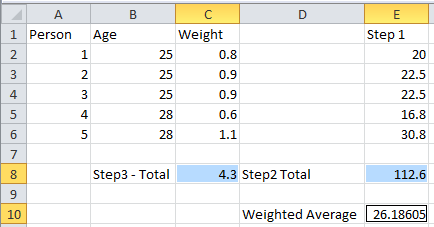# QlikView Documents

Documents for QlikView related information.

Announcements
Qlik Analytics Tour 2020 Online. Begins August 10th. Register Today

# What’s a Weighted Average and how do you calculate it in QlikView?Creator

## What’s a Weighted Average and how do you calculate it in QlikView?

Citing Investopedia, the definition of a ‘Weighted Average’ is “An average in which each quantity to be averaged is assigned a weight. These weightings determine the relative importance of each quantity on the average. Weightings are the equivalent of having that many like items with the same value involved in the average.

Weighted Average.  In Formula, Financial Theory.  Retrieved February 12th, 2014, from http://www.investopedia.com/terms/w/weightedaverage.asp.

I created the below example in Excel to describe the concept:

Assume you have the following data where you have 5 people, 3 of them are 25 years old and 2 are 28 years old.  You then have weightings assigned to the various values:Step 1 - You would the multiple each value by its weight.  To do this in the example, first multiply each value (Age) by it’s weight (Age * Weight):Step 2 – Get the total value of all the numbers calculated in Step1.Step 3 – Add up the total weighting valuesStep 4 – Divide the total value of Step 2 by the total weight of Step 3:This number now represents the weighted average.

To perform this same operation in QlikView, you would create a Chart – a Straight Table.

The Dimension would be on the Age

You would then create 2 Expressions – 1 Expression to calculate the Weighted Total over Age and a final Expression that would calculate the Weighted Average.  You would use the AGGR() function to perform this task – this function will return a valule of an expression calculated over a dimension (ie. Get me the total value of Weight calculated over the Dimension Age)

To create the total for the Weight, you would use the following syntax:

Weighted Total

Sum(Aggr(Sum(Total Weight), Age))

To create the Weighted Average, you would use the following calculation:

Sum(Total Age * Aggr(Sum(Weight), Age)) / Sum(Total Aggr(Sum(Weight), Age))

Tags (2)
Labels (2)

• ### New to QlikViewCreator III

Why you have used the Total key word while calculating the sum of Weight.

In which case we use Total key word and also what is the difference between sum(Weight) and sum(Total Weight).

thank you.Creator
 If the word TOTAL occurs before the function arguments, the calculation is made over all possible values given the current selections, and not just those that pertain to the current dimensional value, that is, it disregards the chart dimensions.

Sum - chart function ‒ QlikViewContributor III

Hi There,

Are you sure you got this right? I mean the in STEP3. I would say you are supposed to divide it by the actual number of occurrences (number of rows) and not by the weight. You have already took the weight into consideration already by calculating new values. Those new values should be simply averaged now.

Version history
Revision #:
1 of 1
Last update:
‎2014-02-24 06:57 PM
Updated by: